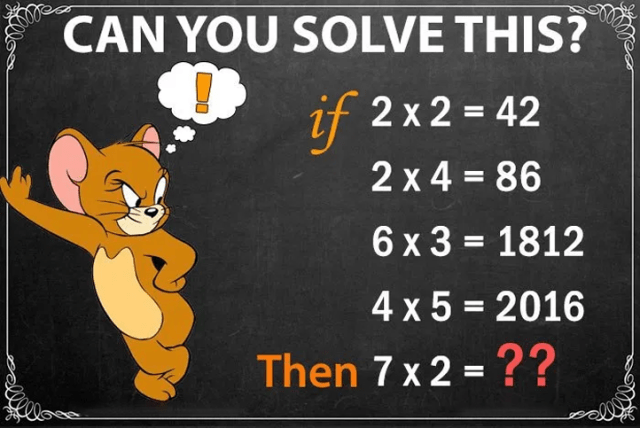# If 2×2=42, Then 7×2=? – Genius Math Puzzles with Answer

Are you able to solve this puzzle?

Math Puzzles for Genius – Only for Genius with Answers

Another easy yet logic-filled arithmetic problem awaits you.

Solve this number series by figuring out the rationale behind this problem calculation.

IF 2×2=42, 2×4=86, 6×3=1812, 4×5=2016, THEN 7×2=__??Isn’t it simple?

.

.

.

.

.

.

147; Logic: First multiply the numbers and then subtract the first digit from the answer i.e 7×2=14, now subtract first digit of LHS from 14; i.e. 14 – 7 = 7, therefore we get 147.